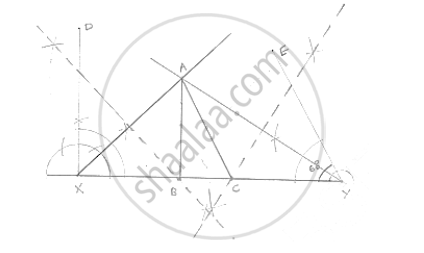# Construct a Right-angled Triangle Whose Perimeter is Equal to 10 Cm and One Acute Angle Equal to 60°. - Mathematics

Construct a right-angled triangle whose perimeter is equal to 10 cm and one acute angle equal to 60°.

#### SolutionSteps of construction:
Step 1: Draw a line segment XY of 10cm.
Step 2: Draw ∠DXY =∠B = 90°and ∠FYX = ∠C = 60°
Step 3: Draw the angle bisectors of∠ DXY  and ∠EYX which intersects each other at A.
Step 4: Draw the perpendicular of AX and AY which intersect XY at B and C respectively.
Step 5: Join AB and AC
∴ Δ ABC is the required triangle

Concept: Some Constructions of Triangles
Is there an error in this question or solution?

#### APPEARS IN

RD Sharma Mathematics for Class 9
Chapter 16 Constructions
Exercise 16.3 | Q 5 | Page 18

Share